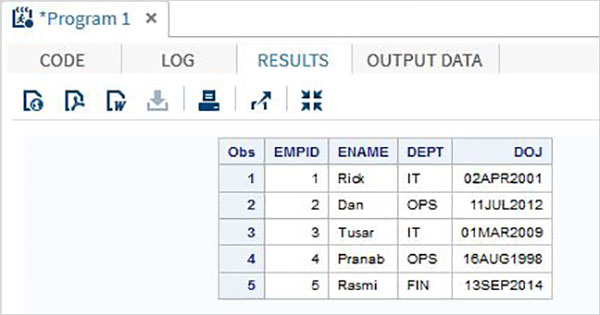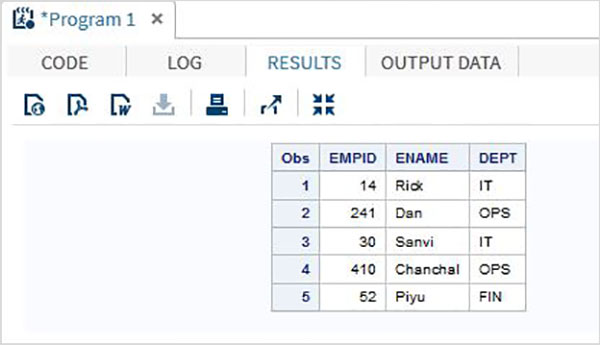# SAS - Input Methods

The input methods are used to read the raw data. The raw data may be from an external source or from in stream datalines. The input statement creates a variable with the name that you assign to each field. So you have to create a variable in the Input Statement. The same variable will be shown in the output of SAS Dataset. Below are different input methods available in SAS.

• List Input Method
• Named Input Method
• Column Input Method
• Formatted Input Method

The details of each input method is described as below.

## List Input Method

In this method the variables are listed with the data types. The raw data is carefully analysed so that the order of the variables declared matches the data. The delimiter (usually space) should be uniform between any pair of adjacent columns. Any missing data will cause problem in the output as the result will be wrong.

### Example

The following code and the output shows the use of list input method.

```DATA TEMP;
INPUT   EMPID ENAME \$ DEPT \$ ;
DATALINES;
1 Rick  IT
2 Dan  OPS
3 Tusar  IT
4 Pranab  OPS
5 Rasmi  FIN
;
PROC PRINT DATA = TEMP;
RUN;
```

On running the bove code we get the following output.## Named Input Method

In this method the variables are listed with the data types. The raw data is modified to have variable names declared in front of the matching data. The delimiter (usually space) should be uniform between any pair of adjacent columns.

### Example

The following code and the output show the use of Named Input Method.

```DATA TEMP;
INPUT
EMPID= ENAME= \$ DEPT= \$ ;
DATALINES;
EMPID = 1 ENAME = Rick  DEPT = IT
EMPID = 2 ENAME = Dan  DEPT = OPS
EMPID = 3 ENAME = Tusar  DEPT = IT
EMPID = 4 ENAME = Pranab  DEPT = OPS
EMPID = 5 ENAME = Rasmi  DEPT = FIN
;
PROC PRINT DATA = TEMP;
RUN;
```

On running the bove code we get the following output.## Column Input Method

In this method the variables are listed with the data types and width of the columns which specify the value of the single column of data. For example if an employee name contains maximum 9 characters and each employee name starts at 10th column, then the column width for employee name variable will be 10-19.

### Example

Following code shows the use of Column Input Method.

```DATA TEMP;
INPUT   EMPID 1-3 ENAME \$ 4-12 DEPT \$ 13-16;
DATALINES;
14 Rick     IT
241Dan      OPS
30 Sanvi    IT
410Chanchal OPS
52 Piyu     FIN
;
PROC PRINT DATA = TEMP;
RUN;
```

When we execute above code, it produces following result −## Formatted Input Method

In this method the variables are read from a fixed starting point until a space is encountered. As every variable has a fixed starting point, the number of columns between any pair of variables becomes the width of the first variable. The character '@n' is used to specify the starting column position of a variable as the nth column.

### Example

The following code shows the use of Formatted Input Method

```DATA TEMP;
INPUT   @1 EMPID \$ @4 ENAME \$ @13 DEPT \$ ;
DATALINES;
14 Rick     IT
241 Dan      OPS
30 Sanvi    IT
410 Chanchal OPS
52 Piyu     FIN
;
PROC PRINT DATA = TEMP;
RUN;
```

When we execute above code, it produces following result −